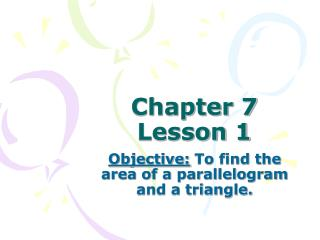Download PresentationChapter 7 Lesson 1Chapter 7 Lesson 1 - PowerPoint PPT Presentation

Download PresentationChapter 7 Lesson 1
An Image/Link below is provided (as is) to download presentation

Download Policy: Content on the Website is provided to you AS IS for your information and personal use and may not be sold / licensed / shared on other websites without getting consent from its author. While downloading, if for some reason you are not able to download a presentation, the publisher may have deleted the file from their server.

- - - - - - - - - - - - - - - - - - - - - - - - - - - E N D - - - - - - - - - - - - - - - - - - - - - - - - - - -
Presentation Transcript

1. Chapter 7 Lesson 1 Objective: To find the area of a parallelogram and a triangle.

2. Theorem 7-1 Area of a Rectangle The area of a rectangle is the product of its base and height. A = bh h b

3. Theorem 7-2 Area of a Parallelogram The area of a parallelogram is the product of a base and the corresponding height. A = bh

4. A base of a parallelogram is any of its sides. The corresponding altitude is a segment perpendicular to the line containing that base drawn from the side opposite the base. The height is the length of an altitude.

5. Example 1: Finding the Area of a Parallelogram Find the area of each parallelogram.

6. Example 2: Finding the Area of a Parallelogram Find the area of a parallelogram with base 12 m and height 9 m. A=bh A=(12)(9) A=108m2

7. Example 3: Finding Area in the Coordinate Plane Finding Area in the Coordinate Plane Find the area of parallelogram PQRS with vertices P(1, 2), Q(6, 2), R(8, 5), and S(3, 5). Graph parallelogram PQRS. If you choose      as the base, then the height is 3.

8. Example 4: Finding Area in the Coordinate Plane Find the area of parallelogram EFGH with vertices E(–4, 3), F(0, 3), G(1, –2), and H(–3, –2). 4 4 2 A=bh A=(4)(5) A=20 units2 5 -4 -2 2 4 -2 -4

9. Example 5: Finding a Missing Dimension For parallelogram ABCD, find CF to the nearest tenth. First, find the area of parallelogram ABCD. Then use the area formula a second time to find CF.

10. A diagonal divides any parallelogram into two congruent triangles. Therefore, the area of each triangle is half the area of the parallelogram.

11. Theorem 7-3  Area of a Triangle The area of a triangle is half the product of a base and the corresponding height. A = ½bh A base of a triangle is any of its sides. The corresponding height is the length of the altitude to the line containing that base.

12. Example 6: Finding the Area of a Triangle Find the area of the shaded triangle. The area of the shaded triangle is 32 ft2

13. Example 7: Finding the Area of a Triangle Find the area of the triangle. A= ½ bh A= ½ (12)(5) A= 30cm2

14. Example 8: Real World Connection Find the area of the figure. triangle area = ½bh =½(20)6 = 60 ft2 rectangle area = bh = 20(12) = 240 ft2 area of the side = 60 + 240 = 300 ft2

15. Assignment